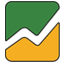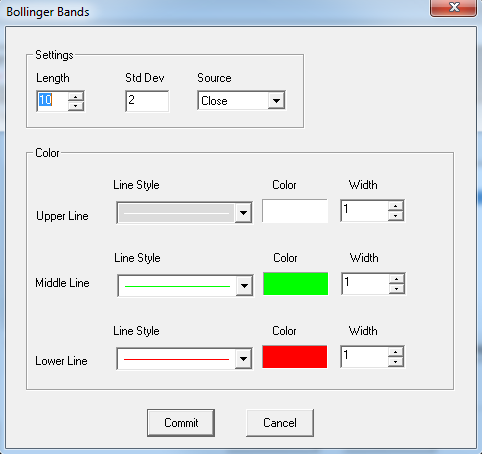# Bollinger Bands

User can add Bollinger Bands indicator to their chart by right click the chart and select Study Config to open the study configure window first, then select the “Bollinger Bands” from the study list and add it into the Studies In Chart field.Bollinger Bands Configure window allows user to modify the Bollinger Bands chart, such as change the study period and lines’ color. User can open this Bollinger Bands Configure window either by click the “Config” button or double click the study “Bollinger Bands” under Studies In Chart section.

## Explanation of Each Field

·         Settings section allows user to modify some study parameters for the Bollinger Bands study.

Ø  Length: Enter the study period/length for Bollinger Bands study.

Ø  Std Dev: Enter the period for standard deviation.

Ø  Source: User can use the dropdown menu to change the study source for the Bollinger Bands study.

§  Open: Use the open price data to calculate the Bollinger Bands.

§  High: Use the highest price data to calculate the Bollinger Bands.

§  Low: Use the lowest price data to calculate the Bollinger Bands.

§  Close: Use the close price data to calculate the Bollinger Bands.

§  (High+Low)/2: Use this equation to calculate the price first, then use those price data to calculate the Bollinger Bands.

§  (High+Low+Close)/3: Use this equation to calculate the price first, then use those price data to calculate the Bollinger Bands.

§  (Open+High+Low+Close)/4: Use this equation to calculate the price first, then use those price data to calculate the Bollinger Bands.

(Hint, those Open, High, Low, and close are represents each bar’s open price, highest price, lowest price, and close price.)

·         Color section allows user to modify the associate field’s line style, line color, and line width.